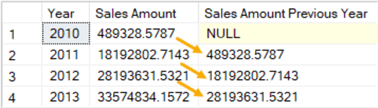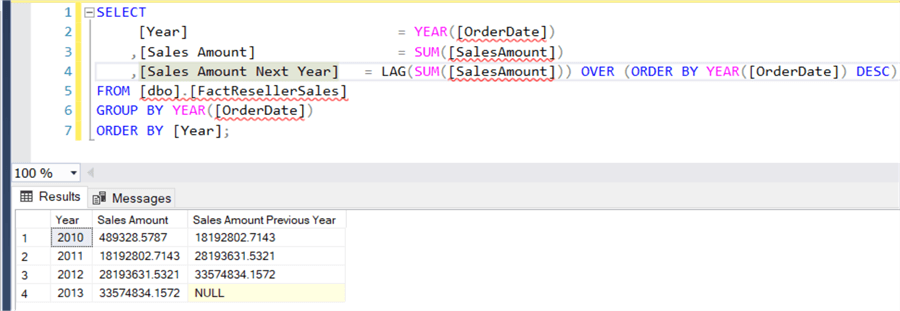# SQL Server Window Functions LEAD and LAG

##### Overview

In this part of the tutorial, we’ll look at offset functions, especially the LAG and LEAD functions. They were introduced in SQL Server 2012 and they made writing specific patterns in T-SQL much easier. With those functions, complex queries using self-joins or cursors can be replaced with easier queries.

The LAG function has the ability to fetch data from a previous row, while LEAD fetches data from a subsequent row. Both functions are very similar to each other and you can just replace one by the other by changing the sort order.

Using the AdventureWorks data warehouse, we’ll calculate the sales amount of the previous year.

```SELECT
[Year]                         = YEAR([OrderDate])
,[Sales Amount]                 = SUM([SalesAmount])
,[Sales Amount Previous Year]   = LAG(SUM([SalesAmount])) OVER (ORDER BY YEAR([OrderDate]))
FROM [dbo].[FactResellerSales]
GROUP BY YEAR([OrderDate])
ORDER BY [Year];
```

We can see that for each year, the data of the previous year has been fetched in the second column:The LAG/LEAD function has also two optional parameters:

• The offset. The default is 1, but you can jump back more rows by specifying a bigger offset. You cannot specify a negative value.
• A default value. When there is no previous row (in the case of LAG), NULL is returned. You can see this in the screenshot in the first row. You can specify a default value to be returned instead of NULL.

If we would sort descending in the window function, LAG will fetch the next row’s value instead of the previous one:To show you the contrast, this is how the previous year values needed to be calculated before LAG/LEAD were introduced:

```WITH CTE_Years AS
(
SELECT
[Year]                         = YEAR([OrderDate])
,[Sales Amount]                 = SUM([SalesAmount])
FROM [dbo].[FactResellerSales]
GROUP BY YEAR([OrderDate])
)
, CTE_PY AS
(
SELECT
y1.[Year]
,y1.[Sales Amount]
,[Sales Amount Previous Year] = y2.[Sales Amount]
FROM CTE_Years y1
LEFT JOIN CTE_Years y2 ON ([y2].[Year] + 1) = [y1].[Year]
)
SELECT * FROM [CTE_PY]
ORDER BY [Year];
```

As you can see, the query is a bit more elaborate since an extra step needs to be taken: first the sales amount per year needs to be calculated, then the results need to be joined to itself in order to fetch the data from the previous year.

#### Calculating Year-over-Year growth

Using LAG, it’s easy to calculate the year-over-year growth of sales. Let’s reuse the query from the previous example:

```WITH CTE_PY AS
(
SELECT
[Year]                         = YEAR([OrderDate])
,[Sales Amount]                 = SUM([SalesAmount])
,[Sales Amount Previous Year]   = LAG(SUM([SalesAmount]))
OVER (ORDER BY YEAR([OrderDate]))
FROM [dbo].[FactResellerSales]
GROUP BY YEAR([OrderDate])
)
SELECT
[Year]
,[Sales Amount]
,[Sales Amount Previous Year]
,[YoY Growth]                   = 100.0 * ([Sales Amount] - [Sales Amount Previous Year])
/ [Sales Amount Previous Year]
FROM [CTE_PY]
ORDER BY [Year];
```

This gives us the following result:## FIRST_VALUE and LAST_VALUE

Both functions are straight forward: they either return the first or the last value of an ordered set. Let’s illustrate using the sample data introduced in the previous part of the tutorial:

```SELECT
[Group]
,[Value]
,FirstValue    = FIRST_VALUE([Value]) OVER (PARTITION BY [Group] ORDER BY [Value])
,LastValue     = LAST_VALUE([Value])  OVER (PARTITION BY [Group] ORDER BY [Value])
FROM [dbo].[Test];
```

The results:The FIRST_VALUE function returns nicely the first value for each group. The LAST_VALUE function however suffers from the same issue as the MAX function in part 4 of the tutorial: it returns the last value of the current frame, which is giving the ascending sort the same value as the current row. If you want to find the maximum value, you better use the MAX function with a descending sort or specify a correct frame extent (ROWS BETWEEN UNBOUNDED PRECEDING AND UNBOUNDED FOLLOWING for example).